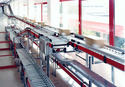## Putting Math to Work

Type of Unit: Problem Solving

# Prior Knowledge

Students should be able to:

• Solve problems with rational numbers using all four operations.
• Write ratios and rates.
• Use a rate table to solve problems.
• Write and solve proportions.
• Use multiple representations (e.g., tables, graphs, and equations) to display data.
• Identify the variables in a problem situation (i.e., dependent and independent variables).
• Write formulas to show the relationship between two variables, and use these formulas to solve for a problem situation.
• Draw and interpret graphs that show the relationship between two variables.
• Describe graphs that show proportional relationships, and use these graphs to make predictions.
• Interpret word problems, and organize information.
• Graph in all quadrants of the coordinate plane.

# Lesson Flow

As a class, students use problem-solving steps to work through a problem about lightning. In the next lesson, they use the same problem-solving steps to solve a similar problem about lightning. The lightning problems use both rational numbers and rates. Students then choose a topic for a math project. Next, they solve two problems about gummy bears using the problem-solving steps. They then have 3 days of Gallery problems to test their problem-solving skills solo or with a partner. Encourage students to work on at least one problem individually so they can better prepare for a testing situation. The unit ends with project presentations and a short unit test.

Subject:
Mathematics
Level:
Middle School
6
Tags:
• Algebra
• Word Problems
• Unit 7 Putting Math to Work•••••••••